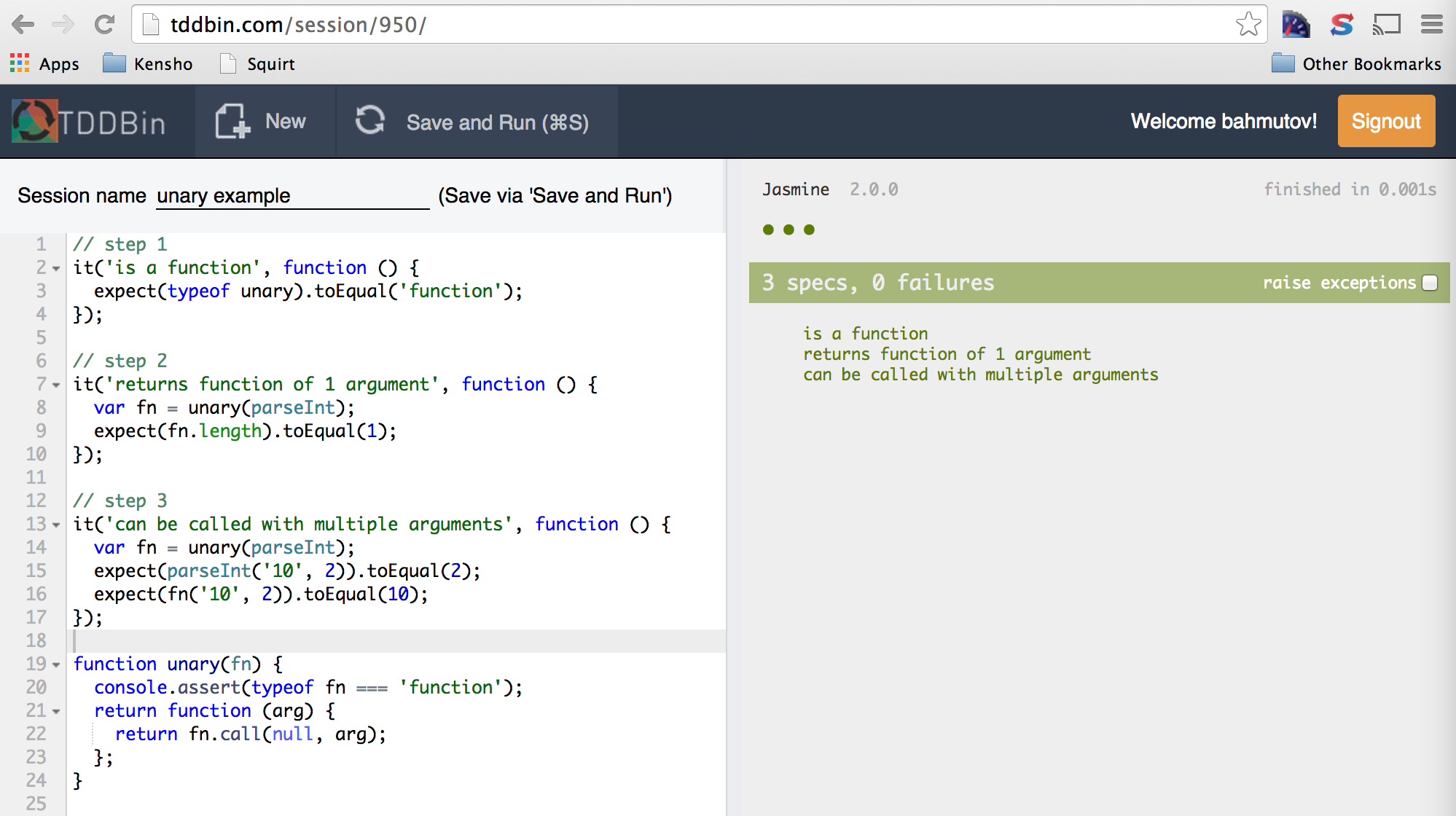# Functional JavaScript interview question

## Writing a functional adaptor makes a nice interview question.

I love using functional programming where possible, and have written several blog posts with examples. These examples make perfect interview questions. They are small, easy to state, and allow the candidate to show real-world code, rather than an abstract design. In this blog post I would like to show that functional questions also have two properties that make them a better judge of a candidate.

• They map naturally to English language, allowing the candidate to express the desired feature before getting bogged down in code.
• Pure functions only depend on their arguments, thus making unit testing them much simpler. Take a look at this interview. The question was to partially apply an `add` function. The candidate started each step of the solution with writing unit tests! This is the best demonstration of test-driven development one can wish for.

The next example is my typical interview question. I will use it to demonstrate how one can solve the problem step by step, then write same solution using JavaScript. I will also show how it can be developed by writing unit tests first.

## Example

This interview question is taken from The Madness of King JavaScript.

step 1: find the problem with this code

The question is pretty simple: why do we get different results when calling `parseFloat` vs `parseInt`?

``````['1', '2', '3'].map(parseFloat);
//=> [1, 2, 3]
['1', '2', '3'].map(parseInt);
//=> [ 1, NaN, NaN ]
``````

I allow the candidate the use computer to experiment and find the answer. I am looking to see if the candidate is familiar with EcmaScript5 array iterators, and in particular with their signatures. Here Array.prototype.map passes each item and its index to the callback function. Next I expect the candidate to look up parseFloat function (takes single string argument) and parseInt function (takes string and radix). At this point I expect the candidate to answer why parseInt produces `NaN` values.

step 2: implement quick fix

After finding the API mismatch, I will ask the candidate to fix this particular array iteration. If the candidate is working on the solution using a laptop, and starts by writing a unit test like below, I consider the candidate a good find

``````function toInts(strings) {
return strings.map(parseInt);
}
it('converts the strings into integers', function () {
expect(toInts(['1', '2', '3'])).toEqual([1, 2, 3]);
});
``````

A typical quick solution for a particular iteration is

``````['1', '2', '3'].map(function (str /*, index, array */) {
return parseInt(str);
});
// [1, 2, 3]
``````

Even when working on white board, I would ask the candidate to verify the solution, and give him/her a laptop. I expect the candidate to type this code into Chrome's DevTools' console, or open Node REPL and try running the code.

`parseInt` expects two arguments, a string and a radix. We can provide value for radix using partial application from the right, or by writing a simple adaptor that only passes first argument to `parseInt`. I will write the following code to give the candidate as a programming goal: implement `unary` function to be used like this

``````['1', '2', '3'].map(unary(parseInt));
// [1, 2, 3]
``````

I expect the candidate to approach this problem by:

• expressing a part of the solution in plain English
• writing an unit test
• coding the part in JavaScript to make unit test pass

Below are my steps to implementing `unary`

step 3 a

English: `unary` is a function that takes a function as its only argument

Test:

``````it('is a function', function () {
expect(typeof unary).toEqual('function');
});
``````

Code:

``````function unary(fn) {
console.assert(typeof fn === 'function');
}
``````

step 3 b

English: `unary` returns another function that only expects a single argument

Test:

``````it('returns function of 1 argument', function () {
var fn = unary(parseInt);
expect(fn.length).toEqual(1);
});
``````

Code:

``````function unary(fn) {
console.assert(typeof fn === 'function');
return function (arg) {
// nothing yet
};
}
``````

step 3 c

English: Returned function can be called with many arguments, but returns the original function called with the first argument only

Test:

``````it('can be called with multiple arguments', function () {
var fn = unary(parseInt);
expect(parseInt('10', 2)).toEqual(2);
expect(fn('10', 2)).toEqual(10);
});
``````

Code:

``````function unary(fn) {
console.assert(typeof fn === 'function');
return function (arg) {
return fn.call(null, arg);
};
}
``````

## Update

A great way to conduct the interview using English - test - code iteration is by using TDDBin. You can write both production and unit test code in the same editor and it keeps running Jasmine. The above example would look like this## Conclusion

In elementary school mathematics classes our teacher always said "Show your work". Functional coding interview questions have a nice property: you can show your work by first expressing what you are trying to achieve in plain English, then writing an unit test, and then coding the solution. The result as you can see is almost 1 to 1 mapping from plain English prose to JavaScript code.